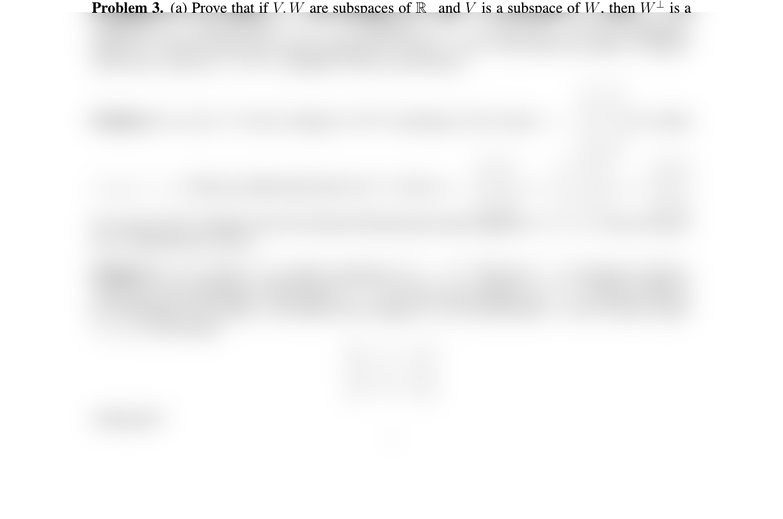Study Guides (380,000)
US (220,000)
UCLA (3,000)
MATH (300)
All (20)
Final

MATH 33AH Lecture Notes - Lecture 13: Orthonormality, Qr Decomposition, Glossary Of Video Game TermsExam

Department
Mathematics
Course Code
MATH 33AH
Professor
All
Study Guide
Final

This preview shows half of the first page. to view the full 2 pages of the document.MATH 33A/2 PRACTICE MIDTERM 2
Problem 1. (True/False) ...
Problem 2. Let v1=
1
1
0
, v2=
1
0
1
, v3=
1
1
1
.
(a) Show that B= (v1, v2, v3)is a basis for R3.
(b) Let u=
1
1
2
. Find the coordinates of uwith respect to the basis B.
(c) Find the change of basis matrix from the standard basis to B.
(d) Let T:R3R3be the reﬂection about the plane x+y+z= 0. Find the matrix of Twith
respec to B.
(e) Let T:R3R3be a linear transformation. Show that Tis invertible if and only if B=
(u1, u2, u3), where u1=T v1,u2=T v2and u3=T v3, is a basis for R3.
(f) With the same notation as in (e), assume that Tis invertible. Express the change of basis matrix
from the standard basis to the basis B.
Problem 3. (a) Prove that if V, W are subspaces of Rnand Vis a subspace of W, then Wis a
subspace of V. Prove that Rn=V+Vand that VV= 0. (b) Let VR5be a subspace,
and let Wconsist of the zero vector and also all vectors win R5that make the angle 30 degrees
Problem 4. (a) Let Vbe the subspace of R4consisting of all vectors v=
x
y
z
w
for which
x+y+z=w. Find an orthonormal basis for V. (b) Let v1=
1
2
3
,v2=
1
2
3
,v3=
2
0
6
be vectors in R3. Explain why the Gramm-Schmidt procedure applied to (v1, v2, v3)does not give
you 3 orthonormal vectors.
Problem 5. (a) A matrix Ais called symmetric if A=AT. Find all 2×2symmetric matrices
which are also orthogonal. What about 3×3? (b) Give an example of a 3×3matrix which is
not orthogonal, but which is invertible and compute its QR factorization. (c) For which scalars
c1, c2, c3is the matrix
1 2 3
4c1c2
5 6 c3
orthogonal?
1